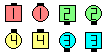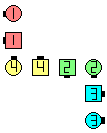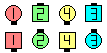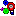Definitions of Square Dance Calls and ConceptsIndex -->  Plus  |  A1  |  A2  |  C1  |  C2  |  C3A  |  C3B  |  C4  |  NOL  |
Definitions (Text Only) -->  Plus  |  A1  |  A2  |  C1  |  C2  |  C3A  |  C3B  |  C4  |  NOL  |
 Find call:

 \$B8@8l(B\$BJ?9T\$J(B Two-Faced Lines \$B\$^\$?\$O(B Promenade (\$B\$3\$N>l9g\$O(B, \$B;X<(\$5\$l\$??M\$,(B Leaders \$B\$NLr\$r\$7\$^\$9(B) \$B\$+\$i!%(B

Leaders \$B\$O30B&\$N?M\$r(B pivot point \$B\$K\$7\$F(B Cast Off 1/4 (Out Anchor 1/4) and Roll \$B\$r\$7\$F(B, Press Ahead \$B\$r\$7(B, Trailers \$B\$O(B 1/2 Circulate \$B\$r\$7\$F(B, Cross Fire \$B\$r\$7\$^\$9!%(B

\$BJ?9T\$J(B Wave \$B\$G=*\$o\$j\$^\$9!%(BLink Up\$B\$NA0(B Leaders Out Anchor 1/4 as Trailers 1/2 Circulate\$B\$N8e(B Centers Cross Fire as Outsides Roll & Press Ahead\$B\$N8e(B (\$B=*\$o\$j(B)

\$B%R%s%H(B: Promenade \$B\$+\$i\$O(B, \$B;X<(\$5\$l\$?%+%C%W%k(B (Leaders \$B\$NLr\$r\$9\$k?M(B) \$B\$,(B, \$BJI\$N@5LL\$r8~\$/\$^\$G(B Promenade \$B\$rB3\$1(B 1/2 Press Ahead \$B\$r\$7(B, \$BB>\$N?M\$O8e\$m\$KIU\$\$\$FJ?9T\$J(B Two-Faced Line \$B\$r:n\$j(B, \$B\$=\$l\$+\$iA40w\$G(B Link Up \$B\$r\$7\$^\$9!%\$3\$N\$h\$&\$KMY\$k\$3\$H\$G(B, \$BJI\$r8~\$\$\$?(B formation \$B\$G=*\$o\$k\$3\$H\$,J]>Z\$5\$l\$^\$9!%(B

\$BCmCast A Shadow, Link Up, Wheel The Ocean | Sea \$B\$,\$"\$j\$^\$9!%(B

\$B\$^\$?(B, \$B

Link Up But anything (Linking anything) [C4] : \$BJ?9T\$J(B Two-Faced Line \$B\$+\$i!%(B Leaders \$B\$O(B Link Up \$B\$r\$7\$F(B, Trailers \$B\$O(B Step Ahead \$B\$r\$7(B, \$B%3%s%Q%/%H\$J(B formation ("normalize \$B\$H\$\$\$\$\$^\$9(B") \$B\$r:n\$j(B, anything \$B\$N%3!<%k\$r\$7\$^\$9!%(B \$B\$3\$l\$O(B Busy anything [C3B] \$B\$HF1\$8\$h\$&\$J;H\$o\$lJ}\$G\$9!%(B

direction Anchor fraction [C4]: From a Couple. Using the dancer nearest the given direction as the pivot point, Cast Off the given fraction toward the given direction. The pivot dancer starts and ends on the same floor spot. There is neither a default direction nor a default fraction for this call.Animation for Link UpCALLERLAB definition for Link UpChoreography for Link UpComments? Questions? Suggestions?